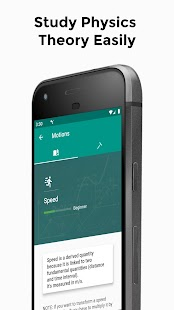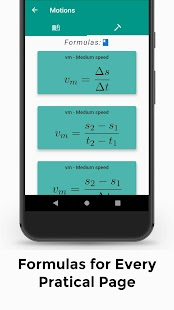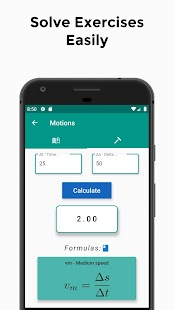PhysicsMaster

Hello Physics student! Introducing PhysicsMaster Pro, the app that allows you to solve your physics exercises.

PhysicsMaster Pro allows you to acquire the basics of Physics and solve problems thanks to the CALCULATOR function on the practical pages of the app!
The Calculator allows you to solve your exercises and checks:
– Enter your data (speed, acceleration, etc..)
– Tell us what you want to find (space, time, etc. …)
– Calculate… And in one click you will have the solution to your exercise (FORMULA and RESULT).

Here are the topics in PhysicsMaster Pro:
– Fundamental Quantities
– Quantities Derived
– Scalar sizes
– Vector sizes
– Measurements and Errors
– Systematic and Random Errors
– Random Errors
– Introduction to Kinematics
– Average and instantaneous speed
– Average and instantaneous acceleration
– Uniform Straight Motorcycle
– Uniformly accelerated motion
– Uniform Circular Motion
– Harmonic Motorcycle
– Introduction to the principles of Dynamics
– First Principle of Dynamics
– Second Principle of Dynamics
– Third Principle of Dynamics
– Introduction to Forces
– Balance
– Motion of a Bullet
– Moment of a Force
– Friction Forces
– Strength-weight
– Motorcycle Satellites
– Apparent Forces
– Harmonic Motorcycle of a Spring
– Work of a Force
– Power
– Motorcycle Quantity
– Kinetic Energy
– Potential Energy
– Laws of Kepler
– Law of Universal Gravitation
– Density
– Pressure
– Introduction: Thermodynamics
– Temperature and Heat
– Thermal Dilatation
– The Perfect Gas
– Changes in the State of Matter
– Principles of Thermodynamics
– Introduction – Wave
– Waves Types
– Periodic wave
– Sound wave
– Doppler effect
– Introduction – Optics
– The Light
– Reflection and refraction
– Lenses
– The electric charge
– Conservation of electrical charge
– Conductors and insulators
– Law of Coulomb
– Dielectric constant
– Electric Field
– Electric Current
– Electrical Resistance
– Laws of Ohm
– Electric Circuit
– Electromagnetic waves

Here are the PRACTICAL PAGES in PhysicsMaster Pro:
– Average and instantaneous speed
– Average and instantaneous acceleration
– Uniform Straight Motorcycle
– Uniformly accelerated motion
– Uniform Circular Motion
– Harmonic Motorcycle
– Balance
– Moment of a Force
– Friction Forces
– Strength-weight
– Motorcycle Satellites
– Harmonic Motorcycle of a Spring
– Work of a Force
– Power
– Motorcycle Quantity
– Kinetic Energy
– Potential Energy
– Law of Universal Gravitation
– Density
– Thermal Dilatation

NEXT RELEASES: QUIZ OF PHYSICS and NEW PRACTICAL PAGES

Integrate your Physics notes with formulas and theory in PhysicsMaster Pro – Basic Physics. It’s the ideal application for college and high school students.
Learn Basic Physics and solve your exercises with ease!
PhysicsMaster Pro is waiting for you!

Let Physics be with you and your students!
Available in Italian, English and Spanish.

What’s New:
* The calculator function is on the front line!
* New contents!

Screenshots

••••••••••••••••••